Glide Planes (a, b, c, n, d)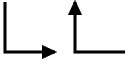The symbols above show glide planes parallel to the plane of the screen, the arrow indicating the glide direction. (Note that for non-orthogonal axes, the angle formed by the symbol is the same as the cell angle rather than 90°.) When present, these symbols are shown at the bottom left-hand corner of the space-group diagram. A fractional value next to the symbol indicates the height of the glide above the XY plane.

The written symbols for glide planes, "a", "b", and "c", indicate the glide direction. An a-glide plane perpendicular to the c-axis and passing through the origin, i.e. the plane x,y,0 with a translation 1/2 along a, will have the corresponding symmetry operator 1/2+x,y,-z.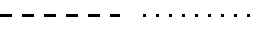The symbols shown above correspond to glide planes perpendicular to the plane of the screen with their normals perpendicular to the dashed/dotted lines. Dashed lines indicate that the glide is parallel to the plane of the screen and along the line while dotted lines indicate that the glide is perpendicular to the plane of the screen.

Thus a space-group diagram for an ab-plane projection will show a c-glide plane perpendicular to the b-axis as a dotted line. If the plane passes through the origin, i.e. the plane is x,0,z, then the corresponding symmetry operator is x,-y,1/2+z.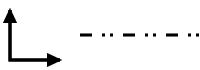In A-, B-, C-, and F-centred space groups, the combination of a centred lattice with a glide plane can lead to a situation where the glide direction exists as one of two possible directions, e.g. a plane perpendicular to say the c-axis may be either an a- or a b-glide plane.

This situation is shown by the symbols above for planes parallel and perpendicular to the plane of the screen, respectively. Note the difference between the dashed and double-dotted lines (indicating either dashed or dotted for the glide direction) with the dashed-dotted line described below.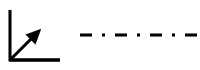The dashed-dotted line shown on the above right indicates a glide plane perpendicular to the XY plane where the glide direction is simultaneously both parallel and perpendicular to the XY plane (in contrast to the dashed double-dotted line described previously). Thus the glide is in a diagonal direction. The equivalent diagonal-glide plane parallel to the plane of the screen is shown in the above left-hand symbol.

Where the translation is 1/2 along each axis direction, the plane is given the written symbol "n". An n-glide plane perpendicular to the c-axis and passing through the origin, i.e. the plane x,y,0 with translations 1/2 along both a and b, will have the corresponding symmetry operator 1/2+x,1/2+y,-z.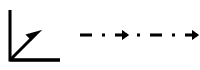A dashed-dotted line with arrows shown on the above right indicates a glide plane perpendicular to the XY plane where the glide direction is simultaneously both parallel and perpendicular to the XY plane, but with a translation only half that of the n-glide plane describe previously. The sense of the translation is indicated by the arrows.

The equivalent d-glide plane parallel to the plane of the screen is shown in the above left-hand symbol. (Note the non-conventional use of a half-arrow!). In this case, the sense of the translation is in the direction of the arrow and along the positive Z direction.

The presence of a d-glide plane automatically implies a centred lattice.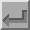Back
 © Copyright 1995-2006.  Birkbeck College, University of London. Author(s): Jeremy Karl Cockcroft